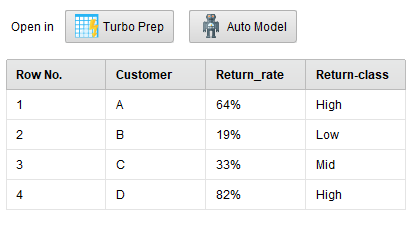# Return numbers

Member Posts: 3Newbie
Hi,
I want to name a return number of ">60% = High" and a return number of "<20% = Low". How can I generate this in the results? I just have the customer ID and the average returns in % for each customer.

Thanks for help!

• Moderator, RapidMiner Certified Analyst, Member Posts: 1,195Unicorn
Hi @marcel_drewes,

I'm not sure to understand  :
">60%" of what ?
Can you describe your dataset and explain (by giving an example) what you want to obtain ?
Depending on what you want to do, maybe Generate Attribute and/or Aggregate operator(s) can be helpful....

Regards,

Lionel
• Member Posts: 3Newbie
Hi,

I am sorry, I am new here. First let me describe my current excel file, it looks like the following:

Customer:__Return rate:__
A_________64%________
B_________19%________
C_________33%________
D_________82%________
...

and I want to convert it into this:

Customer:__Return rate:__(Return-Class:)
A_________64%________(High Returns)
B_________19%________(Low Returns)
C_________33%________(Mid Returns)
D_________82%________(High Returns)
...

so:
>60% = high
<20% = low
21-59% = mid

Thanks for help!
• Moderator, RapidMiner Certified Analyst, Member Posts: 1,195Unicorn
Hi @marcel_drewes,

Here an other example of process which performs what you want to do :
```<?xml version="1.0" encoding="UTF-8"?><process version="9.1.000-BETA2">
<context>
<input/>
<output/>
<macros/>
</context>
<operator activated="true" class="process" compatibility="9.1.000-BETA2" expanded="true" name="Process">
<parameter key="logverbosity" value="init"/>
<parameter key="random_seed" value="2001"/>
<parameter key="send_mail" value="never"/>
<parameter key="process_duration_for_mail" value="30"/>
<parameter key="encoding" value="SYSTEM"/>
<process expanded="true">
<operator activated="true" class="operator_toolbox:create_exampleset" compatibility="1.7.000" expanded="true" height="68" name="Create ExampleSet" width="90" x="45" y="85">
<parameter key="generator_type" value="comma_separated_text"/>
<parameter key="number_of_examples" value="100"/>
<parameter key="use_stepsize" value="false"/>
<list key="function_descriptions"/>
<list key="numeric_series_configuration"/>
<list key="date_series_configuration"/>
<list key="date_series_configuration (interval)"/>
<parameter key="date_format" value="yyyy-MM-dd HH:mm:ss"/>
<parameter key="input_csv_text" value="Customer,Return_rate&#10;A,64%&#10;B,19%&#10;C,33%&#10;D,82%"/>
<parameter key="column_separator" value=","/>
<parameter key="parse_all_as_nominal" value="false"/>
<parameter key="decimal_point_character" value="."/>
<parameter key="trim_attribute_names" value="true"/>
</operator>
<operator activated="true" class="generate_attributes" compatibility="9.1.000-BETA2" expanded="true" height="82" name="Generate Attributes" width="90" x="313" y="85">
<list key="function_descriptions">
<parameter key="Return-class" value="if(parse(replace(Return_rate,&quot;%&quot;,&quot;&quot;))&gt;60,&quot;High&quot;,if(parse(replace(Return_rate,&quot;%&quot;,&quot;&quot;))&lt;20,&quot;Low&quot;,&quot;Mid&quot;))"/>
</list>
<parameter key="keep_all" value="true"/>
</operator>
<connect from_op="Create ExampleSet" from_port="output" to_op="Generate Attributes" to_port="example set input"/>
<connect from_op="Generate Attributes" from_port="example set output" to_port="result 1"/>
<portSpacing port="source_input 1" spacing="0"/>
<portSpacing port="sink_result 1" spacing="0"/>
<portSpacing port="sink_result 2" spacing="0"/>
</process>
</operator>
</process>
```
The results are like that :Hope it helps,

Regards,

Lionel

• Member Posts: 323Unicorn
edited December 2018
• Member Posts: 3Newbie
This task is done. Thanks a lot!• Moderator, RapidMiner Certified Analyst, RapidMiner Certified Expert, Member Posts: 1,635Unicorn
Discretize by User Specification would be another simple way to solve this problem in RapidMiner.
Brian T.
Lindon Ventures
Data Science Consulting from Certified RapidMiner Experts
• Member Posts: 323Unicorn
Thanks @Telcontar120. I just tried it. This is a really straight forward way that I had not seen before.